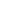# Free CBSE Class 10 Mathematics UNIT III: Coordinate Geometry Coordinate geometry Worksheets

Download free printable Coordinate geometry Worksheets to practice. With thousands of questions available, you can generate as many Coordinate geometry Worksheets as you want.

## Sample CBSE Class 10 Mathematics UNIT III: Coordinate Geometry Coordinate geometry Worksheet Questions

1.

The line segment joining the points A(3,2) and B(5,1) is divided at the point P in the ratio 1:2 and P lies on the line 3x-18y + k =0. Find the value of K.

2.

Find the point on the x-axis which is equidistant from the points (5,4) and (-2,3). Also find the area of triangle formed by points.

3.

Determine the ratio in which the straight line x-y-2=0 divides the line segment joining (3,-1) and (8,9).

4.

Find the ratio in which (-3, q) divides the line segment joining the points (-5,-4) and (-2, 3). Find the value of q.

5.

Prove that the points P(0,-1), Q(-2,3) R(6,7) and S(8,3) are the vertices of a rectangle PQRS.

6.

Prove that the points (7,10) (-2,5) and (3, -4) are the vertices of an isosceles right triangle.

7.

The vertices of triangle PQR are P(6,-2), Q(0,-6) and R(4,8). Find the coordinates of mid point of PQ, QR and PR.

8.

Find the point on y axis which is equidistant from the point (5,-2) and (-3,2).

9.

Find the ration in which (11,15) divides the line segment joining (15,5) and (9,20).

10.

Prove that the diagonals of a rectangle PQRS with vertices P(2,-1), Q(5,1), R(5,6) and S(2,6) are equal and bisect each other.

## Find more Coordinate geometry Worksheets

Worksheets by UrbanPro

Our worksheets are designed to help students explore various topics, practice skills and enrich their subject knowledge, to improve their academic performance. Designed by Experts who have extensive experience and expertise in teaching a subject, these worksheets will improve your child's problem-solving skills and subject knowledge in a fun and interactive manner.
Check out our free customized worksheets across school boards, grades, subjects and levels of subject knowledge. You can download, print and share these worksheets with anyone, anywhere, anytime!

Get a custom worksheet to practice!

Select your topic & see the magic.

subjectSelect Chapter(s)

Chapters & Subtopics